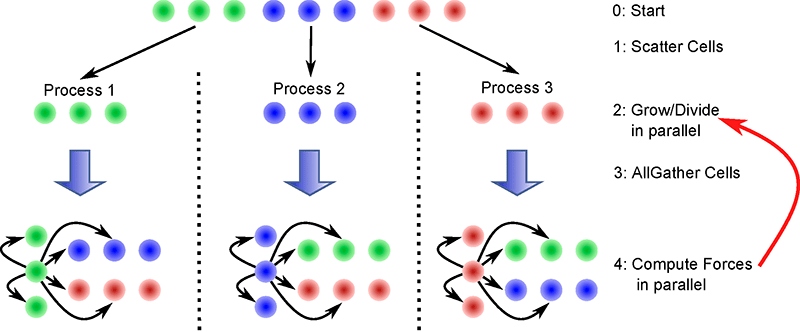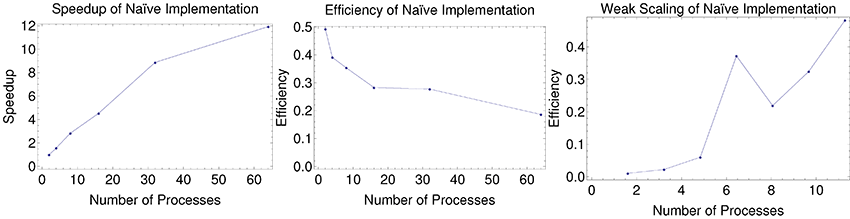# Parallel Simulation of Multicellular Ensembles

Here is a final project for my High Performance Computing Course (CS 205) that I did with Tarik Moon. It’s a continuation of my work with the Arcak Group.

## Background/Motivation:

An interesting idea that has been thrown around in the synthetic biology community is engineering a platform for synthetic multicellularity. The end goal is to eventually be able to inoculate an environment with a bacterial culture, and have the cells grow up, divide, and differentiate into colonies with some sort of spatial organization that we can program to accomplish tasks. The main challenge is one of symmetry: if all of the cells are identically programmed, how do we get the colony to break the symmetry and have distinct fates? Also, cells can only communicate locally, and have to still be able to get a bearing on their overall position within the colony. Control theory and dynamics have allowed applied mathematicians to show certain schemes are capable of achieving certain patterns, but these derivations require the assumption that cells are not growing or moving, which obviously are not true in a living organism. On the other hand, in vivo and in vitro experiments take quite a considerable amount of time and physical resources, with the risk that the platform might not work at all. In silico simulations presents a viable opportunity for synthetic biologists to rapidly prototype their proposed platforms and predict key behavior before deciding to commit to the lab. Furthermore, an in silico simulation would allow for biologists to test certain hypotheses to see if their theory can explain observed experimental behavior, granting both explanatory and predictive power.

## Challenges

The main issue is that we are guaranteed to have exponential time complexity in the problem. This is because the cells divide and the population grows exponentially over time. Unless we can do O(n) updates in O(log n) time (which would probably get us very rich), our problem becomes exponentially slower over time. As a result, paralleling the updates to the cells will be very attractive.

## Approach

### Modeling Basics

Here, we try to choose a reasonably expensive calculation that is only local but would be relevant within a biological context. We chose to implement a basic physics and growth engine to model cells that were growing out in a petri dish. We assume that cells are cylindrical and that their volume is growing exponentially. Once they reach a certain radius that we draw from a Gaussian Distribution, the cell divides, where its radius is reset to 1 and then a new child is spawned with a small displacement in a random direction. Once there are two cells that are overlapping, they push each other with a spring force that is proportional to the difference between the sum of their radii and their distance to each other. Their velocity is proportional to the force, which is a useful assumption under high friction conditions. This gives us the crowding and growth behavior that looks qualitatively right.

### Naive Parallel and Serial Implementation:

Our update algorithm consists of three major phases:

1) Have all of the cells grow and divide

2) Compute the force of each of the cells

3) Update the positions based on the forces

(1) and (3) are both clearly embarrassingly parallel. For (2), we first approach the problem as any other n-body problem. The main premise is that the forces that are computed on each cell are independent for each cell, so that means that we can compute them on individual processes. We implement the parallelization in MPI, and the process is summarized in the figure belowHowever, each cell is looking at all of the other cells, which adds a considerable number of calculations that are not necessary. While tree codes try to get around this by grouping agents that are far apart into one entity, since our interactions are only local, we try another approach with BFS.

### BFS Based Implementation:

We implemented a BFS based algorithm that works the following way:

1) It updates the graph (the adjacency list of each node) in each step

2) While running BFS it checks serially if at each if found any cell adjacent to the cell at distance d. If not, then the process stops, Otherwise the process continues and tries to find the adjacent cells at distance d+1.

The key assumption we made here was that the cells are connected, and that the graph of interactions does not change much between iterations. We traverse through the graph using BFS. So if the connectivity is preserved then new adjacency list for each of the nodes will can be found traversing through the neighbors of the neighbors. And due to the topology of the cells if we do not for any cell that is in the adjacency list for the updated position of the cell we can safely assume that the more distant cells from the cell will not be in the updated adjacency list. This is a pretty safe assumption, except the case when the cells divide. Because in that case the cell size shrinks to 1. To compensate for that we add a threshold value.

### Threshold Value:

Although all the cells divide at a random radius, we know of that maximum radius at the birth of the cell. So if r_max is the maximum of the threshold radius of any of the cells that divided in any stage, we add (r_max  – 1) to the radius of a cell when we are trying to compute the neighbors of that cell. That way we make sure that even though a connected cell got disconnected by dividing, in the graph it remains connected. This way we successfully use the BFS for updating the graph.

## Results

Because of the fact that our cells are growing exponentially and that there are O(n^2) comparisons to be made, our algorithm is compute-bound. As we increase the number of processes for a constant problem size, we see that the efficiency falls down to about a constant 30%, and that our speed up begins to cap out at around 12x. However, as we increase the number of iterations, the efficiency increases quadratically, which means that we have weak-scaling. As a result, it’s quite simple to justify an increase of the number of processes as we increase the problem size. This is because at the beginning, many of the processes don’t have much to do since there aren’t many cells, but the population exponentially grows### Performance Issues with BFS Based Approach:

Although we thought that BFS approach would take a lot lett time than the naive approach, in reality the naive approach was faster. The reason is that in the naive approach we don’t need to construct the relation graphs (as we go through all n elements we can just check the force on the cell by other cells). However, in the case of BFS approach we run BFS n times (once for each cell). So the time complexity is

It turns out that although on average |V|, |E| are are small still  O(|V|+|E|) grows linearly (because as the number of cells increase the cells are more packed and the cell density increases)  i.e.  O(|V|+|E|) ~ O(n)

So the complexity turns out to be very close to . However, here we do extra computation for handling the extra dictionaries and therefore in every case we find that the naive approach is faster than the BFS based approach.

## Future Directions

Perhaps a more efficient way rather than having to search through the tree would be to divide the simulation space into a grid, and assign each process to the cells within a certain grid square. The difficulty with this would be to communicate the geometry of the colony with all of the processes, and then plan out how each process will be able to quickly identify which subset of cells it should be searching through. Furthermore, there are concerns about load balancing, since the cells tend to cluster, and each grid square might have vastly different number of cells.